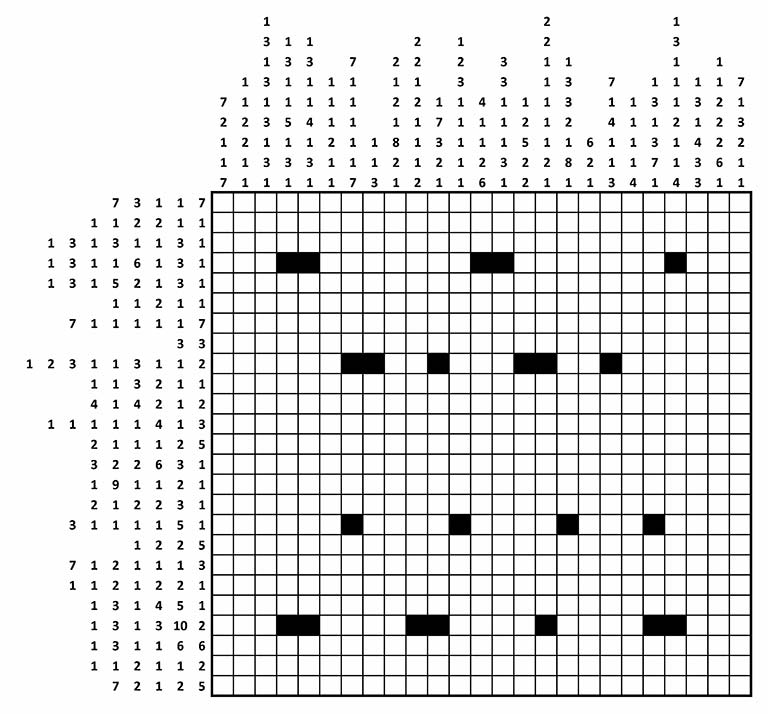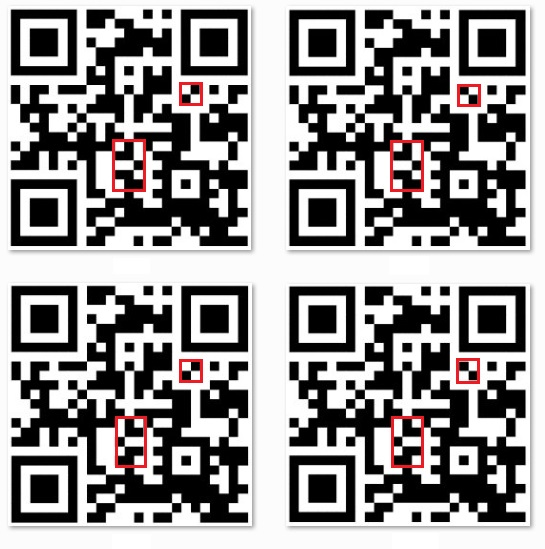The British spy agency GCHQ recently posted a grid-shading puzzle that the director sent out in his Christmas cards this year. The puzzle, shown here, is known as a nonogram and by various other names, including Paint by Numbers and FigurePic:Each cell is to be colored black or white, and the numbers in each row or column indicate the number of cells in each cluster of consecutive black cells, in order. Some of the cells have already been colored black, and it turns out that some of these hints are needed to uniquely solve the puzzle.

In some cases, you can completely solve an entire row or column in isolation, without looking at the rest of the puzzle. For example, row 7 has clue 7 1 1 1 1 1 7, which requires 7 + 1 + 1 + 1 + 1 + 1 + 7 = 19 black cells and at least 7 - 1 = 6 white cells to separate the 7 black clusters. Because that uses up all 25 cells in the row, you can immediately color the whole row: 7 black, 1 white, 1 black, 1 white, and so on.

In other cases, you can only partially solve a row or column in isolation. For example, in row 1, the clue accounts for (7 + 3 + 1 + 1 + 7) + (5 – 1) = 23 cells, leaving a slack of 25 – 23 = 2. So the first cluster of 7 black cells must start no later than column 3, implying that cells in columns 3 through 7 must be black. Similarly, the 3 forces cell (1,11) to be black, and the second 7 forces cells in columns 19 through 23 to be black.

By iterating this type of logic over the rows and columns and using information from other rows and columns, you can solve the whole puzzle by hand. But you can also use mixed integer linear programming (MILP). In 2000, Robert Bosch derived a MILP formulation that I'll use here. The formulation involves three sets of binary decision variables:
\begin{align*} \mathrm{IsBlack}[i,j] &= \begin{cases} 1 & \text{if cell (i,j) is black},\\ 0 & \text{otherwise} \end{cases} \\ \mathrm{IsRowClusterStart}[i,t,j] &= \begin{cases} 1 & \text{if row i, cluster t starts in column j},\\ 0 & \text{otherwise} \end{cases} \\ \mathrm{IsColumnClusterStart}[j,t,i] &= \begin{cases} 1 & \text{if column j, cluster t starts in column i},\\ 0 & \text{otherwise} \end{cases} \end{align*}
For more details, see Bosch's article, where these variables are called $z$, $y$, and $x$, respectively.

You can capture the clues and hints with three SAS data sets:

/* maximum number of clues in rows or columns */ %let rmax = 9; %let cmax = 9;   data row_data; infile datalines missover; input sr1-sr&rmax; i = _N_; datalines; 7 3 1 1 7 1 1 2 2 1 1 1 3 1 3 1 1 3 1 1 3 1 1 6 1 3 1 1 3 1 5 2 1 3 1 1 1 2 1 1 7 1 1 1 1 1 7 3 3 1 2 3 1 1 3 1 1 2 1 1 3 2 1 1 4 1 4 2 1 2 1 1 1 1 1 4 1 3 2 1 1 1 2 5 3 2 2 6 3 1 1 9 1 1 2 1 2 1 2 2 3 1 3 1 1 1 1 5 1 1 2 2 5 7 1 2 1 1 1 3 1 1 2 1 2 2 1 1 3 1 4 5 1 1 3 1 3 10 2 1 3 1 1 6 6 1 1 2 1 1 2 7 2 1 2 5 ;   data column_data; infile datalines missover; input sc1-sc&cmax; j = _N_; datalines; 7 2 1 1 7 1 1 2 2 1 1 1 3 1 3 1 3 1 3 1 1 3 1 1 5 1 3 1 1 3 1 1 4 1 3 1 1 1 1 2 1 1 7 1 1 1 1 1 7 1 1 3 2 1 2 1 8 2 1 2 2 1 2 1 1 1 2 1 7 3 2 1 1 2 3 1 1 1 1 1 4 1 1 2 6 3 3 1 1 1 3 1 1 2 5 2 2 2 2 1 1 1 1 1 2 1 1 3 3 2 1 8 1 6 2 1 7 1 4 1 1 3 1 1 1 1 4 1 3 1 3 7 1 1 3 1 1 1 2 1 1 4 1 3 1 4 3 3 1 1 2 2 2 6 1 7 1 3 2 1 1 ;   data hint_data; input i j; datalines; 4 4 4 5 4 13 4 14 4 22 9 7 9 8 9 11 9 15 9 16 9 19 17 7 17 12 17 17 17 21 22 4 22 5 22 10 22 11 22 16 22 21 22 22 ;

The following PROC OPTMODEL code declares sets and parameters, reads the data, declares the variables and constraints, calls the MILP solver, and outputs the solution to a SAS data set:

proc optmodel; /* declare sets and parameters */ set ROWS; set COLUMNS; set CELLS = ROWS cross COLUMNS; num m = card(ROWS); num n = card(COLUMNS); num rmax = &rmax; num cmax = &cmax; set <num,num> HINTS;   /* cluster-size sequence for row i */ num sr {i in ROWS, t in 1..rmax}; /* cluster-size sequence for column j */ num sc {j in COLUMNS, t in 1..cmax};   /* number of clusters in row i */ num kr {i in ROWS} = card({t in 1..rmax: sr[i,t] ne .}); /* number of clusters in column j */ num kc {j in COLUMNS} = card({t in 1..cmax: sc[j,t] ne .});   /* read clues from data sets */ read data row_data into ROWS=[i] {t in 1..rmax} <sr[i,t] = col(compress('sr'||put(t,best.)))>; read data column_data into COLUMNS=[j] {t in 1..cmax} <sc[j,t] = col(compress('sc'||put(t,best.)))>; read data hint_data into HINTS=[i j];   /* sanity check that sums of cluster sizes agree */ print (sum {i in ROWS, t in 1..kr[i]} sr[i,t]); print (sum {j in COLUMNS, t in 1..kc[j]} sc[j,t]);   /* early and late starts for row i, cluster t */ num er {i in ROWS, t in 1..kr[i]} = if (t = 1) then 1 else (er[i,t-1] + sr[i,t-1] + 1); num lr {i in ROWS, t in 1..kr[i]} = if (t = kr[i]) then (n + 1 - sr[i,kr[i]]) else (lr[i,t+1] - sr[i,t] - 1);   /* early and late starts for column j, cluster t */ num ec {j in COLUMNS, t in 1..kc[j]} = if (t = 1) then 1 else (ec[j,t-1] + sc[j,t-1] + 1); num lc {j in COLUMNS, t in 1..kc[j]} = if (t = kc[j]) then (m + 1 - sc[j,kc[j]]) else (lc[j,t+1] - sc[j,t] - 1);   /* IsBlack[i,j] = 1 if cell (i,j) is black, 0 otherwise */ var IsBlack {CELLS} binary;   /* IsRowClusterStart[i,t,j] = 1 if row i, cluster t starts in column j, 0 otherwise */ var IsRowClusterStart {i in ROWS, t in 1..kr[i], j in er[i,t]..lr[i,t]} binary;   /* IsColumnClusterStart[j,t,i] = 1 if column j, cluster t starts in column i, 0 otherwise */ var IsColumnClusterStart {j in COLUMNS, t in 1..kc[j], i in ec[j,t]..lc[j,t]} binary;   /* declare dummy objective */ min Zero = 0;   /* declare constraints */ con RowSum {i in ROWS}: sum {j in COLUMNS} IsBlack[i,j] = sum {t in 1..kr[i]} sr[i,t];   con ColumnSum {j in COLUMNS}: sum {i in ROWS} IsBlack[i,j] = sum {t in 1..kc[j]} sc[j,t];   con Cluster1Row {i in ROWS, t in 1..kr[i]}: sum {j in er[i,t]..lr[i,t]} IsRowClusterStart[i,t,j] = 1;   con Cluster2Row {i in ROWS, t in 1..(kr[i]-1), j in (er[i,t]+1)..lr[i,t]}: IsRowClusterStart[i,t,j] <= sum {jp in (j+sr[i,t]+1)..lr[i,t+1]} IsRowClusterStart[i,t+1,jp];   con Cluster1Column {j in COLUMNS, t in 1..kc[j]}: sum {i in ec[j,t]..lc[j,t]} IsColumnClusterStart[j,t,i] = 1;   con Cluster2Column {j in COLUMNS, t in 1..(kc[j]-1), i in (ec[j,t]+1)..lc[j,t]}: IsColumnClusterStart[j,t,i] <= sum {ip in (i+sc[j,t]+1)..lc[j,t+1]} IsColumnClusterStart[j,t+1,ip];   con DoubleCoverage1 {<i,j> in CELLS}: IsBlack[i,j] <= sum {t in 1..kr[i], jp in max(er[i,t],j-sr[i,t]+1)..min(lr[i,t],j)} IsRowClusterStart[i,t,jp];   con DoubleCoverage2 {<i,j> in CELLS}: IsBlack[i,j] <= sum {t in 1..kc[j], ip in max(ec[j,t],i-sc[j,t]+1)..min(lc[j,t],i)} IsColumnClusterStart[j,t,ip];   con DoubleCoverage3 {<i,j> in CELLS, t in 1..kr[i], jp in max(er[i,t],j-sr[i,t]+1)..min(lr[i,t],j)}: IsBlack[i,j] >= IsRowClusterStart[i,t,jp];   con DoubleCoverage4 {<i,j> in CELLS, t in 1..kc[j], ip in max(ec[j,t],i-sc[j,t]+1)..min(lc[j,t],i)}: IsBlack[i,j] >= IsColumnClusterStart[j,t,ip];   /* fix variables based on slack in each row or column */ for {i in ROWS, t in 1..kr[i], j in lr[i,t]..(er[i,t]+sr[i,t]-1)} fix IsBlack[i,j] = 1;   for {j in COLUMNS, t in 1..kc[j], i in lc[j,t]..(ec[j,t]+sc[j,t]-1)} fix IsBlack[i,j] = 1;   /* fix variables based on hints */ for {<i,j> in HINTS} fix IsBlack[i,j] = 1;   /* call MILP solver */ solve;   /* create output data set for use by PROC SGPLOT */ create data solution from [i j] IsBlack; quit;

Notice that the PROC OPTMODEL code is completely data-driven so that you can solve other problem instances just by changing the input data sets. In this case, the MILP presolver solves the entire problem instantly:

NOTE: Problem generation will use 4 threads.
NOTE: The problem has 2635 variables (0 free, 169 fixed).
NOTE: The problem has 2635 binary and 0 integer variables.
NOTE: The problem has 6933 linear constraints (2638 LE, 367 EQ, 3928 GE, 0 range).
NOTE: The problem has 24252 linear constraint coefficients.
NOTE: The problem has 0 nonlinear constraints (0 LE, 0 EQ, 0 GE, 0 range).
NOTE: The OPTMODEL presolver is disabled for linear problems.
NOTE: The MILP presolver value AUTOMATIC is applied.
NOTE: The MILP presolver removed all variables and constraints.
NOTE: Optimal.
NOTE: Objective = 0.


Finally, a call to PROC SGPLOT generates a nice plot of the solution:

proc sgplot data=solution noautolegend noborder aspect=1; heatmapparm x=j y=i colorresponse=IsBlack / colormodel=(white black); xaxis display=none; yaxis display=none reverse; run;

The resulting plot is a QR code that leads to further puzzles:If you omit the hints, it turns out that there are four solutions, which you can find by using the CLP solver with the FINDALLSOLNS option:

 /* call CLP solver */ solve with CLP / varselect=fifo findallsolns;   /* create output data set for use by PROC SGPLOT */ create data solution from [s i j]={s in 1.._NSOL_, <i,j> in CELLS} IsBlack[i,j].sol[s];

Here are the four solutions, with the differences marked in red:ShareSenior Manager

Rob Pratt has worked at SAS since 2000 and is a Senior Manager in the Scientific Computing department in the Analytics R&D division. He manages a team of developers responsible for the optimization modeling language and solvers for linear, mixed integer linear, quadratic, and conic optimization. He earned a B.S. in Mathematics (with a second major in English) from the University of Dayton and both an M.S. in Mathematics and a Ph.D. in Operations Research from The University of North Carolina at Chapel Hill.

1.•